## Git First-Parent

February 18, 2018

Messy git history is a display problem, not a data problem.

The first thing I encountered learning about git: there's a lot of conflict about whether it's important to keep a "clean" git history by squashing, rebasing instead of merging, etc. If the --first-parent featue were well supported, it would give us the best of both worlds.

## Regexes for replacing ugly unittest-style assertions

December 30, 2017

In case they help anyone else, here are some regular expressions I used once to convert some ugly unittest-style assertions (e.g. self.assertEqual(something, something_else) to the pytest style (simply assert something == something_else):

(Pytest gives nice informative error messages even if you just use the prettier form.)

Note:

• The option -i means "do it in-place" (modify the file). Including ".bak" means "make backups of the old version with this extension".
• I don't actually want the backups, but (for some odd reason) on my Mac, not asking for them changed how the regex was interpreted to something that's not right.
• After reviewing and checking in the changes I wanted, I cleaned up the backups with git clean -f (careful you don't have any unchecked-in changes you want to keep!).

## An Interaction or Not? How a few ML Models Generalize to New Data

March 4, 2015

Source code for this post is here.

This post examines how a few statistical and machine learning models respond to a simple toy example where they're asked to make predictions on new regions of feature space. The key question the models will answer differently is whether there's an "interaction" between two features: does the influence of one feature differ depending on the value of another.

In this case, the data won't provide information about whether there's an interaction or not. Interactions are often real and important, but in many contexts we treat interaction effects as likely to be small (without evidence otherwise). I'll walk through why decision trees and bagged ensembles of decision trees (random forests) can make the opposite assumption: they can strongly prefer an interaction, even when the evidence is equally consistent with including or not including an interaction.

I'll look at point estimates from:

• a linear model
• decision trees and bagged decision trees (random forest), using R's randomForest package
• boosted decision trees, using the R's gbm package

I'll also look at two models that capture uncertainty about whether there's an interaction:

BART has the advantage of expressing uncertainty while still being a "machine learning" type model that learns interactions, non-linearities, etc. without the user having to decide which terms to include or the particular functional form.

Whenever possible, I recommend using models like BART that explicitly allow for uncertainty.

# The Example

Suppose you're given this data and asked to make a prediction at $X_1 = 0$, $X_2 = 1$ (where there isn't any training data):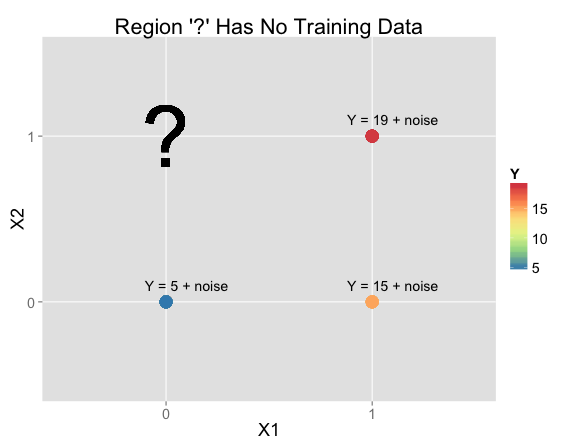X1 X2 Y N Training Rows:
0 0 Y = 5 + noise 52
1 0 Y = 15 + noise 23
1 1 Y = 19 + noise 25
0 1 ? 0

## Covariance As Signed Area Of Rectangles

March 26, 2014

A colleague at work recently pointed me to a wonderful stats.stackexchange answer with an intuitive explanation of covariance: For each pair of points, draw the rectangle with these points at opposite corners. Treat the rectangle's area as signed, with the same sign as the slope of the line between the two points. If you add up all of the areas, you have the (sample) covariance, up to a constant that depends only on the data set.

Here's an example with 4 points. Each spot on the plot is colored by the sum corresponding to that point. For example, the dark space in the lower left has three "positively" signed rectangles going through it, but for the white space in the middle, one positive and one negative rectangle cancel out.

In this next example, x and y are drawn from independent normals, so we have roughly an even amount of positive and negative:

## Formal Explanation

The formal way to speak about multiple draws from a distribution is with a set of independent and identically distributed (i.i.d.) random variables. If we have a random variable X, saying that X1, X2, … are i.i.d means that they are all independent, but follow the same distribution.

## Previous Posts

February 16, 2014

### Simulated Knitting (post)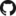I created a KnittedGraph class (subclassing of Python's igraph graph class) with methods corresponding to common operations performed while knitting:

I then embed the graphs in 3D space. Here's a hat I made this way: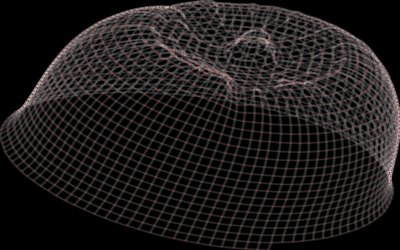### 2D Embeddings from Unsupervised Random Forests (1, 2)There are all sorts of ways to embed high-dimensional data in low dimensions for visualization. Here's one:

1. Given some set of high dimensional examples, build a random forest to distinguish examples from non-examples.
2. Assign similarities to pairs of examples based on how often they are in leaf nodes together.
3. Map examples to 2D in such a way that similarity decreases decreases with Euclidean 2D distance (I used multidimensional scaling for this).

Here's the result of doing this on a set of diamond shapes I constructed. I like how it turned out: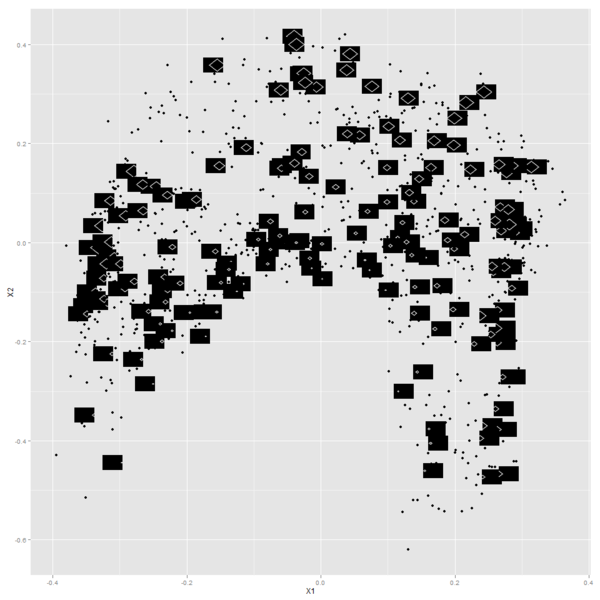### A Bayesian Model for a Function Increasing by Chi-Squared Jumps (in Stan) (post)In this paper, Andrew Gelman mentions a neat example where there's a big problem with a naive approach to putting a Bayesian prior on functions that are constrained to be increasing. So I thought about what sort of prior would make sense for such functions, and fit the models in Stan.

I enjoyed Andrew's description of my attempt: "... it has a charming DIY flavor that might make you feel that you too can patch together a model in Stan to do what you need."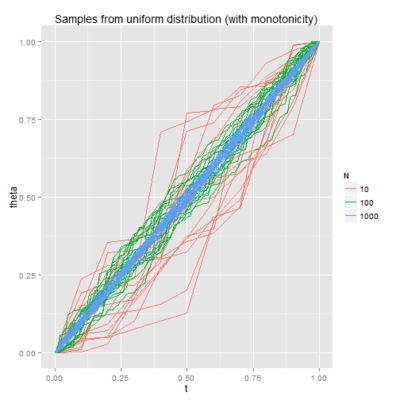### Lissijous Curves JSFiddle

Some JavaScript I wrote (using d3) to mimick what an oscilloscope I saw at the Exploratorium was doing: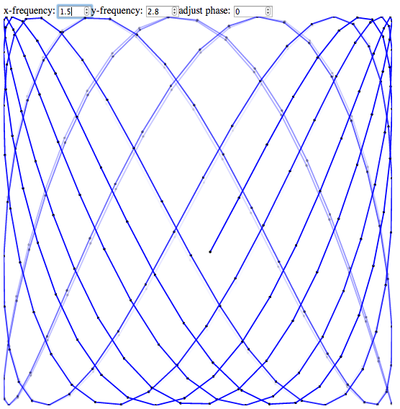### Visualization of the Weirstrass Elliptic Function as a Sum of Terms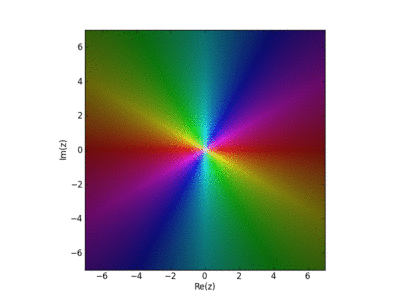John Baez used this in his AMS blog Visual Insight.